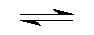## Tetra Aqua Cooper(II+) Cation Complex Hydrolise by Aris Kaksis  Department of medical Biochemistry, RigaStradin University RSU

At equilibrium, the following equilibrium equationis established withits conjugate base:

Cu2+(H2O)4(aq)     +      H2O(l)Cu2+(H2O)3(OH-)(aq)  + H3O+(aq)

acid                                                                  conjugate base
The acid and its conjugate base have different colours. At low pH values the concentration of H3
O+ishigh and
so the equilibrium position lies to the left. The equilibrium solution has the
colour A. At high pH values,the
concentration of H3
O+ is low - the equilibrium position thus lies to the right and the equilibrium solution has  colour B.

Blue (Acid)           pHhydrolize=6           red (Base)

We can apply equilibrium law to Brønsted equilibria - in generalfor a weak acid cooper complex:
Keq=( [H3O+]·[Cu2+(H2O)3(OH-)]/([Cu2+(H2O)4][H2O]))eq
Keq is known as the Brønsted equilibria constant. The pH for hydrolyse is calculated at this point when:
[Cu2+(H2O)3(OH-)] =   [Cu2+(H2O)4]
So from equation: Keq = [H3O+]/[H2O] =10 -7.7/[H2O]= 10 -7.7/55.33 =10 -9.44
Keq = [H3O+]/ [H2O] ; Keq[H2O]=[H3O+]=10-6; -log(Keq[H2O])=6 = pH = -log([H3O+])
The pH of the solution at its equilibrium point is called the pH andis the pH at which half of the cooper is
in its acid form and the other half in the form of its conjugate base.

Aqua complex of cooper
At a low pH<6, a weak acid aqua complex of cooper ion is almostentirely in the [Cu2+(H2O)4]form,the
concentration of which predominates. As the pH increases pH>6- the concentration of [Cu2+(H2O)4]
decreases and the equilibrium is pushed to the right to conjugate base [Cu2+(H2O)3(OH-)] form,the
concentration of which predominates.

We can apply equilibrium law to complexe stability constant equilibria- in general for a acid form cooper cation:
Cu2+(aq)    + OH- (aq)Cu2+(OH-)(aq)
blue (Acid)                                                           red (Base)
Kstab=[Cu2+(OH-)]/([Cu2+][OH-])=106.3=([Cu2+(OH-)][H3O+])/([Cu2+][10-14])
Kstab is known as the Complexe stability constant.The pH for hydrolyse is calculated at this point when:
[Cu2+(OH-)] =   [Cu2+]
So from equation: Kstab = [H3O+]/10-14 =106.3
Kstab10-14=[H3O+]=10-7.7;  -log(Keq10-14)=log(10-7.7) = 7.7 = pH= -log([H3O+])
The pH of the solution at its equilibrium point is called the pH and is the pH at which half of the cooper is in its
conjugate base and the other half in the form of its acid form.

Complex maker of cooper cation
At a low pH<9.44, a weak acid aqua complex of cooper ion isalmostentirely in the Cu2+formfree cation, the
concentration of which predominates. As the pH increasespH>9.44 - the concentration of [Cu2+]decreases and the
equilibrium is pushed to the right to conjugate base [Cu2+(OH-)]form,the concentration of which predominates.

Literature

Handbook of  Chemical Equilibria in Analytical Chemistry.S.Kortly, L.Sucha. New York, Ellis HorwoodLTD. 1985. 414.p.